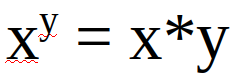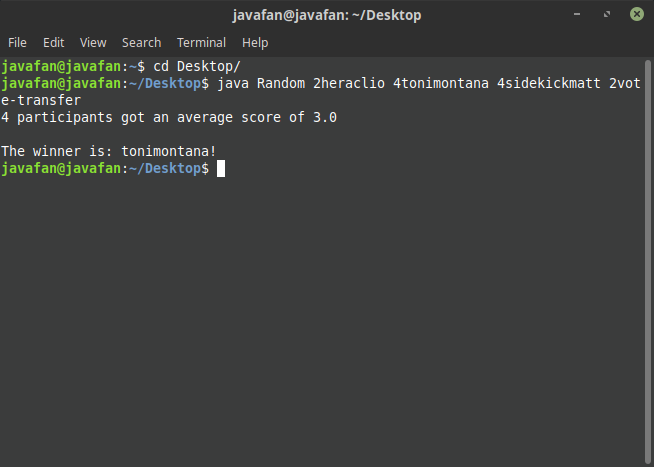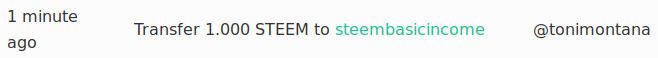# Mathematical SBI contest #4 Results and Solution of the Equation

in #puzzle3 years ago

## Solution:

The problem of the last contest was:The best way to reach the 3 solutions was by thinking about how to eliminate the problem that the y in the exponents brings.
One way to do this was using `y = 1` which simplifies the equation to:
`x = x`
So the first solution is `(x, 1)`.
An other way to eliminate y is to set `x = 0`. That way both sides become zero, if y is bigger than 0(0⁰ is undefined, 1/0 is infinity).
So the second solution is `(0, y); y > 0`.
The last solution, which in my opinion is the most intuitive one can be reached by assuming `x = y`:
`x^x = x*x`
`x^x = x²` |log_x()
`x = 2`
So the last solution is `(2, 2)`

↓↑↓↑↓↑↓↑↓↑↓↑↓↑↓↑↓↑↓↑↓↑↓↑↓↑↓↑↓↑↓↑↓↑↓↑↓↑↓↑↓↑↓↑↓↑↓↑↓

### How your chance of winning is calculated:

• Every participant gets 1 point for entering the contest.
• Every solution entered gets 1 additional point.
• Your winning chance is `"your points"/"total points"`

↓↑↓↑↓↑↓↑↓↑↓↑↓↑↓↑↓↑↓↑↓↑↓↑↓↑↓↑↓↑↓↑↓↑↓↑↓↑↓↑↓↑↓↑↓↑↓↑↓

### List of participants with their scores and chance of winning, sorted by time of entry:

Namesolutions foundscorechance of winning
@heraclio`(2, 2)`216.67 %
@tonimontana`(x, 1)`, `(2, 2)`, `(0, y), y > 0`433.33 %
@sidekickmatt`(2, 1)`, `(2, 2)`, `(0, 1)`, `…`433.33 %
@vote-transfer`(2, 2)`216.67 %

↓↑↓↑↓↑↓↑↓↑↓↑↓↑↓↑↓↑↓↑↓↑↓↑↓↑↓↑↓↑↓↑↓↑↓↑↓↑↓↑↓↑↓↑↓↑↓↑↓

### Winner draw(using a self written program):Congratulations @tonimontana, you won 1 SBI:↓↑↓↑↓↑↓↑↓↑↓↑↓↑↓↑↓↑↓↑↓↑↓↑↓↑↓↑↓↑↓↑↓↑↓↑↓↑↓↑↓↑↓↑↓↑↓↑↓

### The next contest starts tomorrow. Don't miss it!

Sort:

Damn I got lucky there :)